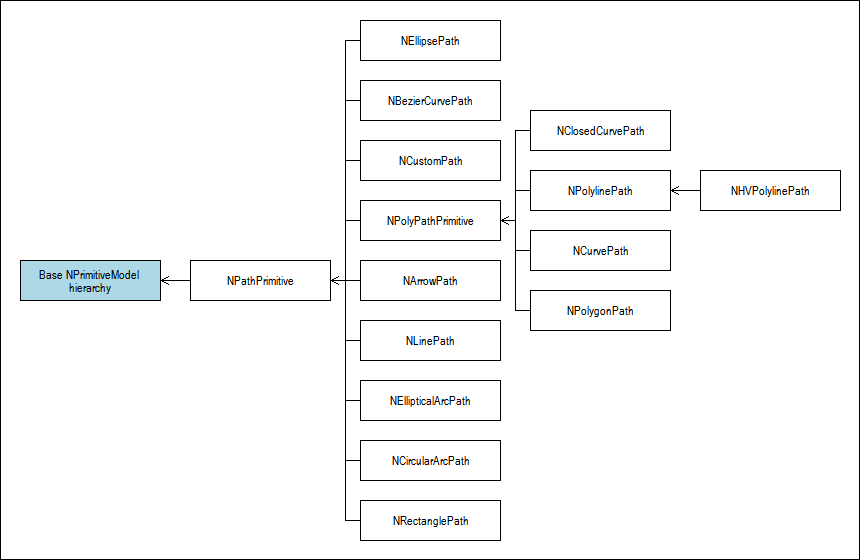﻿ Paths
Nevron .NET Vision Documentation
Paths

Paths are primitive models whose content is a single GDI+ graphics path. The base class for all paths is the abstract NPathPrimitive class, which derives from the NPrimitiveModel class. In order provide an abstraction for all GDI+ primitives, the DOM exposes an extensive set of NPathPrimitive derivates, which can help you display and interactively edit any GDI+ graphics path.

Path primitives use the following class hierarchy:Common Path Features

The NPathPrimitive class adds support for the following common features shared by all paths:

• Path Type - there are only two types of paths - open figures and closed figures. The path type can be obtained from the PathType property (returns a value from the PathType enumeration). Open figure paths can display arrowheads at their start and end points and typically override the ModelStartPoint and ModelEndPoint properties set accessors, to provide control over the model definition of the path start and end points.
• Points - paths consistently expose a set of base and control points via the implementation of the INPoints interface. The exposed points are always in scene coordinates, but alter the path model definition. The particular model aspect modified by a path point is described in the programmers reference for the respective path primitive.

You can always get all points via the Points property get accessor, however the Points property set accessor will by default throw a NotImplementedException. It is up to derived classes to determine whether the bulk points set makes sense for them.

The following example demonstrates some operations you can perform with all paths:
C#
Copy Code
```// get the path points
NPointF[] points = path.Points;

// get only the path points count
int pointCount = path.PointsCount;

// create a bezier curve and alter its first control point
NBezierCurve bezierCurve = new NBezierCurvePath(new NPointF(0, 0), new NPointF(10, 10), new NPointF(20, 0), new NPointF(30, -10));
bezierCurve.SetPointAt(1, new NPointF(10, 30));
```
Visual Basic
Copy Code
```' get the path points
Dim points As NPointF() = path.Points

' get only the path points count
Dim pointCount As Int32 = path.PointsCount

' create a bezier curve and alter its first control point
Dim bezierCurve As New NBezierCurvePath(New NPointF(0, 0), New NPointF(10, 10), New NPointF(20, 0), New NPointF(30, -10))
bezierCurve.SetPointAt(1, New NPointF(10, 30))
```
• Path, Path Points and Path Points Type - the points exposed by the INPoints interface help you modify the path model and may not always be the same as the points of the contained graphics path.

For example: the circular arc exposes three points, which help you modify the circle radius as well as its start and sweep angle, but the points of the graphics path, which is used to display this path are entirely different. On the other hand for a polyline the points and the path points are identical.

NPathPrimitive provides you with several properties, which help you examine the graphics path used to display the path in scene coordinates: PathPathPointsTypes and PathPoints.
• Path Model - paths expose a set of properties beginning with the "Model" prefix, which help you modify different model aspects. Paths also expose a set of DefineModel methods, which help you replace the current model with a new one.
• Flat Paths - it is often required to obtain a flat representation of a path. This however may not always be necessary, because the path can already be flat. You can query whether a path primitive contains a flat graphics path with the help of the IsFlat property. If the path is not flat you can obtain a flat representation with the help of the Flatten methods.
Poly Paths

In addition of the INPoints interface some paths also implement the INPolyPoints interface, which allows you to dynamically insert and remove points from their path model. The root class for all such paths is the NPolyPathPrimitive abstract class. For example:

C#
Copy Code
```// create a polyline with 3 points
NPolylinePath polyline = new NPolylinePath(new NPointF[] {new NPointF(0, 0), new NPointF(10, 10), new NPointF(20, 15)});
// remove the second point
polyline.RemovePointAt(1);
```
Visual Basic
Copy Code
```' create a polyline with 3 points
Dim polyline As New NPolylinePath(New NPointF() {New NPointF(0, 0), New NPointF(10, 10), New NPointF(20, 15)})
' remove the second point
polyline.RemovePointAt(1)
```

The NPolyPathPrimitive class also adds core implementation of the INSegments interface, which allows you to treat each two adjacent points as a single segment. Segments can be split and moved.

Currently Available Paths

The following table summarizes the currently available instancable paths:

Path class Description
NLinePath Represents a line - you can control the start and end points of a line.
NBezierCurvePath Represents a bezier curve - you can control the start, end, first control and second control points of a bezier curve.
NRectanglePath Represents a rectangle - you can control the corners of a rectangle (actually a quadrangle).
NEllipsePath Represents an ellipse - an can control the ellipse X and Y radius, as well as the ellipse model angle.
NCircularArcPath Represents an arc of a circle - the circular arc is defined by three points (start point, end point and control point)
NEllipticalArcPath Represents an arc of an ellipse - the elliptical arc is defined by three points (start point, end point and control point) and two additional parameters - ratio between the X and Y radiuses and angle of the ellipse X-axis).
NCustomPath Represents a custom graphics path
NArrowPath Represents the path of a single or double arrow.
Poly paths
NPolygonPath Represents a polygon - the polygon is defined by a set of points. You can insert and remove points from a polygon.
NClosedCurvePath Represents a closed curve - the closed curve is defined by a set of points and curve tension. You can insert and remove points from a closed curve.
NCurvePath Represents a curve - the curve is defined by a set of points and curve tension. You can insert and remove points from a curve.
NPolylinePath Represents a polyline - the polyline is defined by a set of points. You can insert and remove points from a polyline.
NHVPolylinePath Represents a polyline, which strives to retain the orthogonality of its segments in scene coordinates.